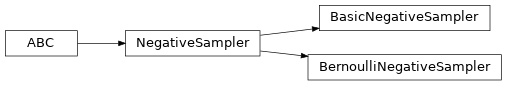# Negative Sampling¶

Because most knowledge graphs are generated under the open world assumption, knowledge graph embedding models must be trained involving techniques such as negative sampling to avoid over-generalization.

Two common approaches for generating negative samples are pykeen.sampling.BasicNegativeSampler and pykeen.sampling.BernoulliBasicSampler in which negative triples are created by corrupting a positive triple $$(h,r,t) \in \mathcal{K}$$ by replacing either $$h$$ or $$t$$. We denote with $$\mathcal{N}$$ the set of all potential negative triples:

$\begin{split}\mathcal{N} &=& \bigcup_{(h,r,t) \in \mathcal{K}} \mathcal{N}(h, r, t)\\ \mathcal{N}(h, r, t) &=& \mathcal{T}(h, r) \cup \mathcal{H}(r, t)\\ \mathcal{T}(h, r) &=& \{(h, r, t') \mid t' \in \mathcal{E} \land t' \neq t\}\\ \mathcal{H}(r, t) &=& \{(h', r, t) \mid h' \in \mathcal{E} \land h' \neq h\}\end{split}$

In theory, all positive triples in $$\mathcal{K}$$ should be excluded from this set of candidate negative triples $$\mathcal{N}$$ such that $$\mathcal{N}^- = \mathcal{N} \setminus \mathcal{K}$$. In practice, however, since usually $$|\mathcal{N}| \gg |\mathcal{K}|$$, the likelihood of generating a false negative is rather low. Therefore, the additional filter step is often omitted to lower computational cost. It should be taken into account that a corrupted triple that is not part of the knowledge graph can represent a true fact.

## Functions¶

 Get the negative sampler class.

## Classes¶

 BasicNegativeSampler(triples_factory[, …]) A basic negative sampler. BernoulliNegativeSampler(triples_factory[, …]) An implementation of the Bernoulli negative sampling approach proposed by [wang2014].

## Class Inheritance Diagram¶## Base Classes¶

class NegativeSampler(triples_factory, num_negs_per_pos=None, filtered=False)[source]

A negative sampler.

Initialize the negative sampler with the given entities.

Parameters
filter_negative_triples(negative_batch)[source]

Filter all proposed negative samples that are positive in the training dataset.

Normally there is a low probability that proposed negative samples are positive in the training datasets and thus act as false negatives. This is expected to act as a kind of regularization, since it adds noise signal to the training data. However, the degree of regularization is hard to control since the added noise signal depends on the ratio of true triples for a given entity relation or entity entity pair. Therefore, the effects are hard to control and a researcher might want to exclude the possibility of having false negatives in the proposed negative triples. Note: Filtering is a very expensive task, since every proposed negative sample has to be checked against the entire training dataset.

Parameters

negative_batch (LongTensor) – The batch of negative triples

Return type

Tuple[LongTensor, Tensor]

hpo_default: ClassVar[Mapping[str, Mapping[str, Any]]]

The default strategy for optimizing the negative sampler’s hyper-parameters

property num_relations

The number of relations to sample from.

Return type

int

abstract sample(positive_batch)[source]

Generate negative samples from the positive batch.

Return type

Tuple[LongTensor, Optional[Tensor]]